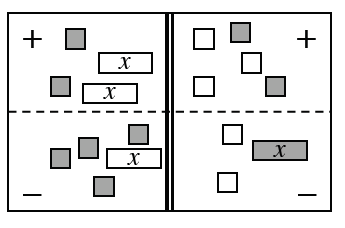### Home > AC > Chapter 2 > Lesson 2.1.9 > Problem2-86

2-86.

Translate the Equation Mat at right into an equation. Remember that the double line represents “equals.”

Write the equation listing all of the tiles on the mat.

$2−2x−(−x+4)=2−3−(x−2)$Use the eTool below to translate the Equation Mat into an equation.
Click the link at right for the full version of the eTool: 2-86 HW eTool